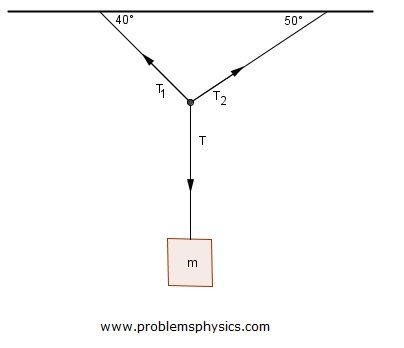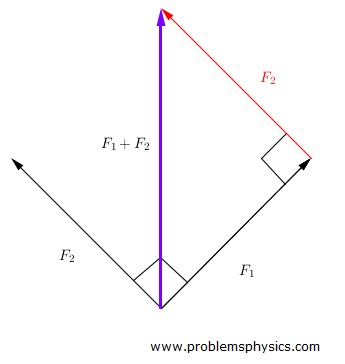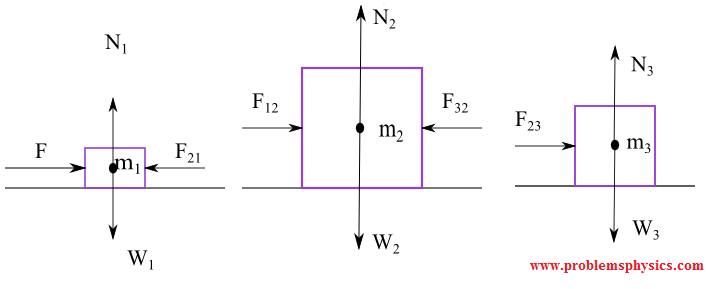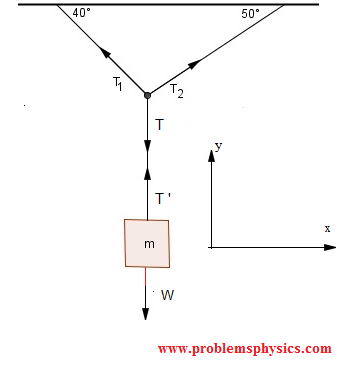# Free SAT II Physics Practice Questions with Solutions Forces and Newton's Laws

Sat Physics subject questions on forces , with detailed solutions at the bottom of the page, similar to the questions in the SAT test are presented. The concepts of forces, inclined planes, tension, free body diagrams, net forces, acceleration, components of a force and Newton's laws are used to solve these questions.
Although with the use of components to represent forces the solutions seems longer than usual , components are used to represent forces because they offer a way to explain the forces acting on a given object without too much ambiguities as to the direction of these forces.

1. What is the magnitude of the friction force exerted by the floor on a $2.5$ Kg mass accelerated by a horizontal force of $25$ Newtons and the magnitude of the acceleration is $4$ $m/s^2$?

2. What is the magnitude of the resultant force due to two forces of 30 Newtons and 40 Newtons acting on an object at a point such that the two forces are at right angle to each other?

3. Two forces $F_1$ and $F_2$ act on a mass m located at the origin of a system of axis x - y and R is the resultant of the two forces. $F_1$ makes an angle of $33^\circ$ with the x axis and has a magnitude of 10 Newtons. $F_2$ makes an angle of $123^\circ$ with the x axis and has a magnitude of 20 Newtons. What angle does the resultant R makes with force $F_1$?

4. An object of mass m rests on a horizontal plane. If we start inclining the plane at an angle $\theta$ with the horizontal, at which angle will the object start moving if the coefficient of friction is equal to 1?

5. As the angle of an incline plane increases, the kinetic force of friction exerted by the incline plane on a mass m:
A) remains the same
B) increases then decreases
C) decreases then increases
D) increases
E) decreases

6. A 2-kilogram box is accelerated by a horizontal force $F$. If the magnitude of the acceleration is $2 m/s^2$ and the force of friction is $4$ N, what is the magnitude of $F$ ?

7. A 10-newton force is pushing $3$ masses $m_1 = 1$ Kg , $m_2 = 6$ Kg and $m_3 = 3$ Kg horizontally on a frictionless floor; see diagram below. What is the magnitude of force exerted by $m_1$ on $m_2$?8. If the weight of an object on a scale in an elevator is more than normal; this means that the elevator must
A) be at rest
B) be moving up
C) have a negative acceleration
D) have a positive acceleration
E) be moving down

9. According to Newton's first law, if a block is pushed on a frictionless floor until it reaches a speed of $0.2 m/s$ then let go, it will
A) move a small distance then stops
B) move a large distance then stops
C) continue moving at the same speed of 0.2 m/s
D) accelerate
E) decelerate

10. Mass $m$ of $10$ Kg is suspended using the three strings system. What are the magnitude of tensions $T_1$ and $T_2$ if mass $m$ is at equilibrium?#### Solutions to Above Questions

1.  A frictional force is in the opposite direction as the accelerating force. If we assume the force of acceleration of $25$ Newtons is in the positive direction then the frictional force $F_r$ will be directed in the negative direction. We now apply Newton's second law Sum of forces acting on a mass m = mass $\times$ acceleration      $W + N + F + Fr = m (|a| , 0)$      ,      |a| is the magnitude of the acceleration W is the weight of the mass and N is the normal force exerted by the floor on the mass. W and N have vertical components only and they are such that N = - W , hence W + N = 0 F and Fr have horizontal components and therefore are written in components form as follows: F = (|F| , 0) and F_r = (-|F_r| , 0). The above vector equation is now written as:      (|F| , 0) + (-|F_r| , 0) = m (|a| , 0) We now use the x-components to write the equation      $25 - |F_r| = 2.5 \times 4$ Solve for $|F_r|$      $|F_r | = 15$ Newtons

2.  Using Pythagora's theorem, the magnitude of the resultant $F_1 + F_2$ is given by: $| F_1 + F_2 | = \sqrt{ (30 N)^2 + (40 N)^2} = 50 N$3.  A diagram of the forces $F_1$, $F_2$ and their resultant $R$ is shown. It is important to note that the angle between $F_1$ and $F_2$ is equal to $123^\circ - 33^\circ = 90^\circ$, and with an angle of $90^\circ$, the calculation of angle $\alpha$ between $F_1$ and $R$ which make a right triangle with $F_2$ is carried out as follows $tan(\alpha)=\dfrac{20}{10}=2$ and $\alpha = arctan(2) \approx 63.4^\circ$4.  The free body diagram below shows all forces acting on the object of mass m: Its weight $W$ where$W = (W_x , W_y) = (-|W|\sin\theta , -|W| \cos\theta)$ $N$ the normal force to the inclined plane and $F_s$ the force of friction exerted by the inclined plane opposite to the motion downward. Force $N$ and the y-component of W have equal magnitude because m cannot move along the y-axis. Hence $|N| = W_y = |W| \cos \theta$ For mass m to start moving downward we need to have the condition: $|W_x| > Fs$ The force of friction is related to the normal force $N$ by: $|F_s| = \mu |N|$ $|W|\sin\theta > \mu |N|$ $|W|\sin\Theta > \mu |W| \cos \theta$ $\tan \theta > 1$ $\theta > 45^{\circ}$

5.  If the weight $W$ of the mass m is decomposed into its components $W_x$ parallel and $W_y$ perpendicular to the incline plane making an angle \theta with the horizontal, then $W_x = |W| sin \theta$ and $W_y = |W| \cos \theta$ The magnitude of force of friction $F_s$ is proportional to the magnitude component perpendicular to the incline plane. $|F_s| = \mu |W_y| = \mu |W| \cos \theta$ Hence as $\theta$ increases, $\cos \theta$ decreases and therefore the magnitude of the force of friction $F_s$ decreases. Answer D.

6.  If $W$ is the weight, $N$ is the normal force exerted by the floor on the box, $F_s$ is the force of friction and $a$ is the acceleration in the same direction as $F$, then according to Newton's second law, we write $W + N + F - F_s = m a$ (in vector form) $W$ and $N$ have equal magnitude and opposite direction, hence W + N = 0 We now write $F$, $F_s$ and $m a$ in components form( x horizontal and y vertical) $F = (|F| , 0)$ , $F_s = (-|F_s| , 0)$ and $a = (|a| , 0)$ Hence $(|F| , 0) + (-|F_s| , 0) = m (|a| , 0)$ $|F| - |F_s| = m |a|$ $|F| = |F_s| + m |a| = 4 + 2 \times 2 = 8$ N

7.  The free body diagram for each mass is shown below. $W_1$ and $N_1$ are the weight and the normal force on $m_1$; $F_{21}$ is the force exerted by $m_2$ on $m_1$ and $F_{12}$ is the force of reaction of $m_1$ on $m_2$ which we want to determine. $F_{32}$ is the force exerted by by $m_3$ on $m_2$ and $F_{23}$ is the force of reaction exerted by $m_2$ on $m_3$.Let a be the acceleration of the three masses in the direction from left to right (in the same direction as F). We now use Newton's second law for each mass to write the following vector equations: $F - F_{21} + W_1 + N_1 = m_1 a$ $F_{12} - F_{32} + W_2 + N_2 = m_2 a$ $F_{23} + W_3 + N_3 = m_3 a$ In each of the equations above, the sum of the weight and the normal force is equal to 0 since there is no movement on the vertical direction; hence the above equation may be simplified and written using the magnitudes only. $|F| - |F_{23}| = m_1 |a|$      (equation 1) $|F_{12}| - |F_{32}| = m_2 |a|$      (equation 2) $|F_{23}| = m_3 |a|$      (equation 3) Forces of action and reaction are equal in magnitude; hence $|F_{21}| = |F_{12}|$ $|F_{23}| = |F_{32}|$ Add the right hand terms and left hand terms of all three equations (1,2 and 3) above and simplify to obtain $|F| = ( m_1 + m_2 + m_3 ) |a|$ $|a| = |F| / (m_1 + m_2 + m_3) = 10 / 10 = 1 m/s^2$ The magnitude of the force exerted by $m_1$ on $m_2$ is $|F_{12}| = m_2 |a| + |F_{32}|$      (using equation 2) $|F_{12}| = m_2 |a| + m_3 |a|$      (using equation 3) $|F_{12}| = m_2 |a| + m_3 |a| = |a| (m_2 + m_3) = 9 N$

8.  The forces acting on an object on a scale on an elevator are its weight $m g$ and the normal force $N$ exerted by the scale on the object. Let us assume that the positive direction is upward; according to Newton's second law, we can write $m a = - m g + N$ $- m g$ is the weight directed downward weight hence the negative sign, and $N$ is positive upward normal force $N$ is the reading of the scale hence the weight of the object in the elevator. $N = m a + mg$ Since $N$ is bigger than $m g$ (the normal weight); it means that $m a$ is positive which also means that the acceleration a is positive. Answer D.

9.  According to Newton's first law, if no net force is acting on the object then it will continue moving at the same speed. In this problem, the only forces acting on the block are the weight and the normal force whose sum is equal to 0. The floor is frictionless. So when the block is pushed then let go the net force acting on the block is zero. Answer C.

10.  The free body diagram of mass $m$ includes the tension of the string $T '$ and the weight $W$ of mass $m$.For equilibrium of mass $m$, according to Newtons second Law, we need to have      $W + T ' = 0$ and $T_1 + T_2 + T = 0$ (vector equations) 1) We first express $W + T ' = 0$ in components form and solve it. In components form, using the system of axes shown in the figure, $W$ and $T '$ may be written as follows:      $W = ( 0 , -|W|)$ and $T ' = (0 , |T '|)$ Using the compoments, the above vector equatiom may be writtwn as      $( 0 , -|W|) + (0 , |T '|) = 0$ y-components give: $-|W| + |T '| = 0$      $|T '| = |W| = m g$, where g $\approx 10 m/s^2$      $|T '| = 100$ N 2) We now express the vectors $T_1$, $T_2$ and $T$ in components forms      $T_1 = (-|T_1| \cos 40^{\circ} , |T_1| \sin 40^{\circ} )$      $T_2 = (|T_2| \cos 50^{\circ} , |T_2| \sin 50^{\circ} )$      $T = ( 0 , |T|)$ Rewrite the vector equation $T_1 + T_2 + T = 0$ using the above component forms to obtain      $(-|T_1| \cos 40^{\circ} , |T_1| \sin 40^{\circ} ) + (|T_2| \cos 50^{\circ} , |T_2| \sin 50^{\circ} ) +( 0 , |T|) = 0$ The x-components give the equation      $-|T_1| \cos 40^{\circ} + |T_2| \cos 50^{\circ} = 0$ The y-components give the equation      $|T_1| \sin 40^{\circ} ) + |T_2| \sin 50^{\circ} ) + |T| = 0$ Solve the above system for $|T_1|$ and $|T_2|$, we obtain      $|T_1| = |T| \cos 50^{\circ}$      $|T_2| = |T| \cos 40^{\circ}$ T and T ' are equal in magnitude because they represent the tension of the same string. Hence      $|T_1| = 100 \cos 50^{\circ}$ N      $|T_2| = 100 \cos 40^{\circ}$ N